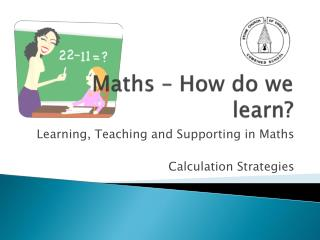DownloadDownload PresentationMaths – How do we learn?

# Maths – How do we learn?

Download Presentation## Maths – How do we learn?

- - - - - - - - - - - - - - - - - - - - - - - - - - - E N D - - - - - - - - - - - - - - - - - - - - - - - - - - -
##### Presentation Transcript

1. Maths – How do we learn? Learning, Teaching and Supporting in Maths Calculation Strategies

2. We teach 4 strands of Maths • Number • Shape, Space and Measures • Data Handling • Using and Applying Maths Using and Applying Maths happens in all 3 other strands, as well as in other lessons, such as Science.

3. Number Today we will look at number. • The four operations (+, -, x and ÷) I will show you the methods that we teach children to use to add, subtract, multiply and divide. We will also look at some of the resources we use to teach number in School.

4. The Four Operations • The four operations in calculations are grouped into Adding and Multiplying – where the answer gets bigger Subtracting and dividing – where the answer gets smaller

5. Number Lines We use number lines in all four operations. We start with numbered lines like this one – 0 1 2 3 4 5 6 7 8 9 10

6. Number lines Then we move on to blank number lines, like this one – Children add on their own numbers to solve calculation problems.

7. Adding Children begin in EYFS and Key Stage 1 by adding real objects, such as cubes. They combine sets and count the total. Count 4 cakes. Count 3 cakes. How many altogether? + =

8. Adding We then move on to using number lines and counting up in ones. 14 + 5 = 19 +1 +1 +1 +1 +1 14 19

9. Partitioning Next we learn to partition – split the number into tens and units – to add. What is 72 + 14? T U 70 2 10 4 70+10= 80 2+4= 6 80+6=86

10. Adding by Partitioning Now we can add by partitioning – 86 + 57 = +50 +4 +3 86 136 140 143

11. Adding by Partitioning Or by rounding then adjusting 754 + 96 (rounding and adjusting) +100 -4 754 850 854

12. Column Addition Finally, we move on to adding in columns, using place value. HTU ThHTU 597 7648 + 475 and + 1486 12 160 9134 9001 1 1 1072

13. Multiplying Again, multiplication begins with drawings and practical activities. How many is three lots of 2? x x x x x x

14. Multiplying Now we use the number line for repeated addition. I have 4 5p coins, how much is that? x1 x2 x3 x4 0 5p 10p 15p 20p

15. Multiplying using arrays 3 x 4 = 12 4 x 3 = 12

16. The Grid Method We can partition to multiply using a grid, like this – 72 x 38 = 70 2 30 2100 60 8 560 16

17. Column Method for x 4346 x 8 48 320 2400 32000 34768

18. Subtracting We begin by ‘taking away’, ‘counting back’ and seeing ‘how many left.’ We made 6 mince pies. We ate 2. How many mince pies are left? x x x x x x

19. Subtracting (number lines) We can use a number line to count back. 22 – 7 = -5 -2 15 20 22

20. Subtracting (number lines) In Key Stage 2, we count UP the number line to find the difference. What is the difference between 34 and 72? +30 +8 34 64 72

21. Subtracting with columns First we use partitioning and subtract hundreds, tens and units. 754 – 286 = H T U 700 + 50 + 4 - 200 + 80 + 6 700 + 40 + 14 - 200 + 80 + 6 600 + 140 + 14 - 200 + 80 + 6 400 + 60 + 8 400 + 60 + 8 = 468

22. Subtracting with ‘exchanging’ 5 13 1 6 4 6 7 - 2 6 8 4 3 7 8 3

23. Dividing Division starts with practical sharing - If I share my 12 sweets between 3 people, how many do they each get?

24. Division with number lines Number lines are also used to count up in division. A baker bakes 24 buns. She puts 6 in every box. How many boxes can she fill? x1 x2 x3 x4 0 6 12 18 24

25. Division with remainders Finally, we can jump in groups and record remainders on the number line. What is 42 divided by 4? 10 groups (Remainders) x10 +1 +1 0 40 41 42

26. A nod to the future... Our School is becoming increasingly excellent at teaching these strategies, but we must now move on to applying what we know. We have recently begun introducing reasoning questions and problem solving tasks into our lessons in order to promote higher order thinking skills (HOTS). Explaining how you know and answering ‘why?’ questions helps us to understand at a deeper level.

27. Future Maths Workshops I would like to discuss reasoning, problem solving and the importance of vocabulary in future sessions. Any other ‘hot’ maths topics?